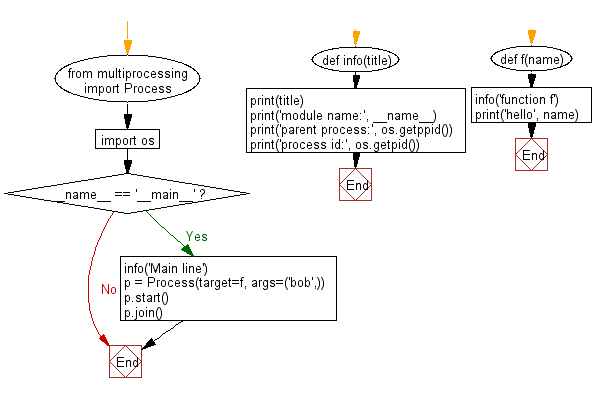﻿ Python: Show the individual process IDs involved - w3resource# Python: Show the individual process IDs involved

## Python Basic - 1: Exercise-118 with Solution

In multiprocessing, processes are spawned by creating a Process object.
Write a Python program to show the individual process IDs (parent process, process id etc.) involved.

Sample Solution:

Python Code:

``````from multiprocessing import Process
import os
def info(title):
print(title)
print('module name:', __name__)
print('parent process:', os.getppid())
print('process id:', os.getpid())
def f(name):
info('function f')
print('hello', name)
if __name__ == '__main__':
info('Main line')
p = Process(target=f, args=('bob',))
p.start()
p.join()
``````

Sample Output:

```Main line
module name: __main__
parent process: 23967
process id: 27986
function f
module name: __main__
parent process: 27986
process id: 27987
hello bob
```

Flowchart:Python Code Editor:

Have another way to solve this solution? Contribute your code (and comments) through Disqus.

What is the difficulty level of this exercise?

Test your Programming skills with w3resource's quiz.

﻿

## Python: Tips of the Day

What is the difference between Python's list methods append and extend?

append: Appends object at the end.

```x = [1, 2, 3]
x.append([4, 5])
print (x)
```

Output:

```[1, 2, 3, [4, 5]]
```

extend: Extends list by appending elements from the iterable.

```x = [1, 2, 3]
x.extend([4, 5])
print (x)
```

Output:

```[1, 2, 3, 4, 5]
```

Ref: https://bit.ly/2AZ6ZFq